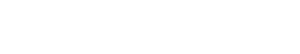# Term frequency count matrix

The term Term frequency count matrix refers to a numerical representation of the number of times a term appears in a document. This matrix can be used to calculate various measures such as inverse document frequency (IDF) and tf-idf. The term frequency count matrix is also known as the bag of words model.

The term Term frequency count matrix may also refer to:

• A measure of how often a given word appears in a text corpus
• The results of a document-term matrix, which can be used as input for several types of statistical models

## Comparing Term frequency count matrix to similar terms

Term frequency (TF) is a measure that reflects how often a given term appears in a text corpus. Inverse document frequency (IDF) is a measure that reflects how rare a given term is across all documents in a text corpus. tf-idf is the product of TF and IDF. Document-term matrix is a matrix that contains the frequencies of terms that occur in documents.

All of these measures are related, but they are not interchangeable. The term “Term frequency count matrix” most accurately refers to the numerical representation of the number of times a term appears in a document. This matrix can be used to calculate various measures such as inverse document frequency (IDF) and tf-idf. The term frequency count matrix is also known as the bag of words model.

When outside of the text analytics industry, the term “Term frequency count matrix” may refer to a measure of how often a given word appears in a text corpus, or the results of a document-term matrix. However, it is important to note that these are not the same as a Term frequency count matrix as used in text analytics.

## Benefits of Term frequency count matrix

There are many benefits of using a Term frequency count matrix when working with text data. This matrix can be used to calculate various measures such as inverse document frequency (IDF) and tf-idf. The term frequency count matrix is also known as the bag of words model.

The term frequency count matrix is a very useful tool for text analytics because it can be used to calculate a variety of measures that are helpful in understanding and analyzing text data. For example, the inverse document frequency (IDF) can be calculated using a Term frequency count matrix. IDF is a measure that reflects how rare a given term is across all documents in a text corpus. This measure is helpful in understanding which terms are more important and should be given more weight when analyzing a text corpus. Additionally, the tf-idf measure can be calculated using a Term frequency count matrix. Tf-idf is the product of TF and IDF. This measure is helpful in understanding which terms are more important and should be given more weight when analyzing a text corpus.

In conclusion, the term Term frequency count matrix refers to a numerical representation of the number of times a term appears in a document. This matrix can be used to calculate various measures such as inverse document frequency (IDF) and tf-idf. The term frequency count matrix is also known as the bag of words model. When outside of the text analytics industry, the term “Term frequency count matrix” may refer to a measure of how often a given word appears in a text corpus, or the results of a document-term matrix. However, it is important to note that these are not the same as a Term frequency count matrix as used in text analytics. There are many benefits of using a Term frequency count matrix when working with text data. This matrix can be used to calculate various measures such as inverse document frequency (IDF) and tf-idf. The term frequency count matrix is also known as the bag of words model.Unlock the power of actionable insights with AI-based natural language processing.